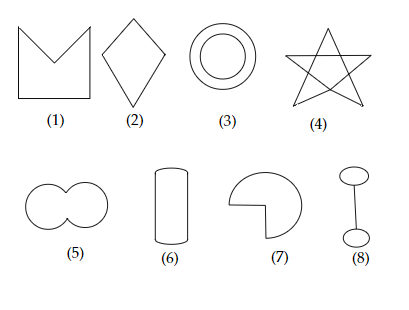# Given here are some figures.Classify each of them on the basis of the following.(a) Simple curve(b) Simple closed curve(c) Polygon(d) Convex polygon(e) Concave polygon."\

To do:

We have to classify each of the given figures on the basis of the following.

(a) Simple curve
(b) Simple closed curve
(c) Polygon
(d) Convex polygon
(e) Concave polygon.

Solution:

We know that,

Simple curves:

Simple curves do not cross or intersect.

Simple closed curve:

Does not have end points and does not intersect.

Polygon:

polygon is a closed plane or two-dimensional figure whose sides are straight line segments.

Convex Polygon:

A polygon is convex if it contains all the line segments connecting any pair of its points.

Concave Polygon:

A polygon that is not convex is said to be a concave polygon.

Therefore,

(a) Figures 1, 2, 5, 6 and 7 are simple curves

(b) Figures 1, 2, 5, 6 and 7 are simple closed curves.

(c) Figures 1 and 2 are polygons

(d) Figure 2 is a convex polygon.

(e) Figure 1 is a concave polygon.